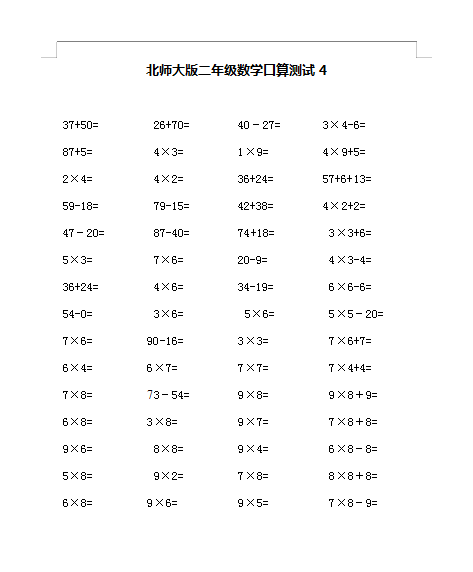37+50=         26+70=        40－27=       3×4-6=

87+5=          4×3=         1×9=         4×9+5=

2×4=          4×2=         36+24=        57+6+13=

59-18=         79-15=        42+38=        4×2+2=       47－20=        87-40=        74+18=         3×3+6=

2022：新北师大版小学数学2年级下册口算 (5)（doc文档2电页子档下载）